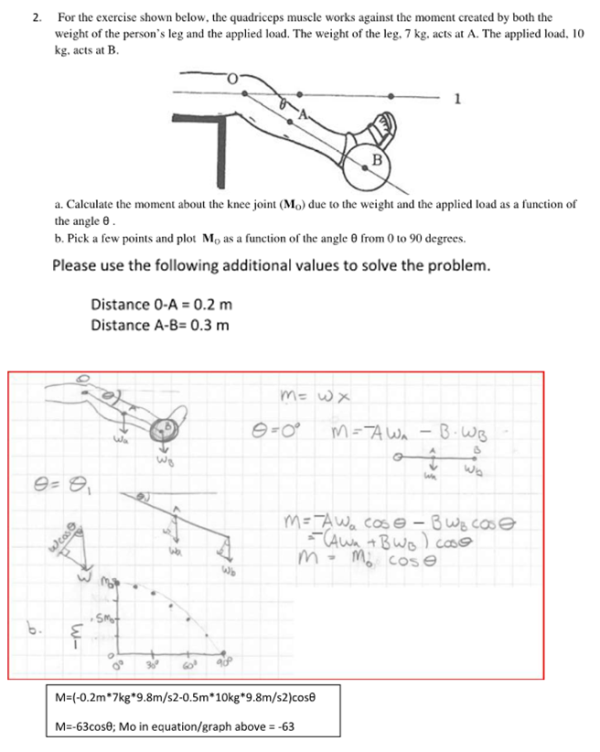For the exercise shown below, the quadriceps muscle works against the moment created by both the weight of the person's leg and the applied load. The weight of the leg, 7 kg, acts at A. The applied load, 10 kg, acts at B. a) Calculate the moment about the knee joint due to the weight and the applied load as a function of the angle theta. b) Pick a few points and plot M0 as a function of the angle from 0 to 90 degress.For the exercise shown below, the quadriceps muscle works against the moment created by both the weight of the person's leg and the applied load. The weight of the leg, 7 kg, acts at A. The applied load, 10 kg, acts at B. a) Calculate the moment about the knee joint due to the weight and the applied load as a function of the angle theta. b) Pick a few points and plot M0 as a function of the angle from 0 to 90 degress.

biomechanics page 1 biomechanics biomechanics biomechanics biomechanics biomechanics biomechanics biomechanics biomechanics page 2